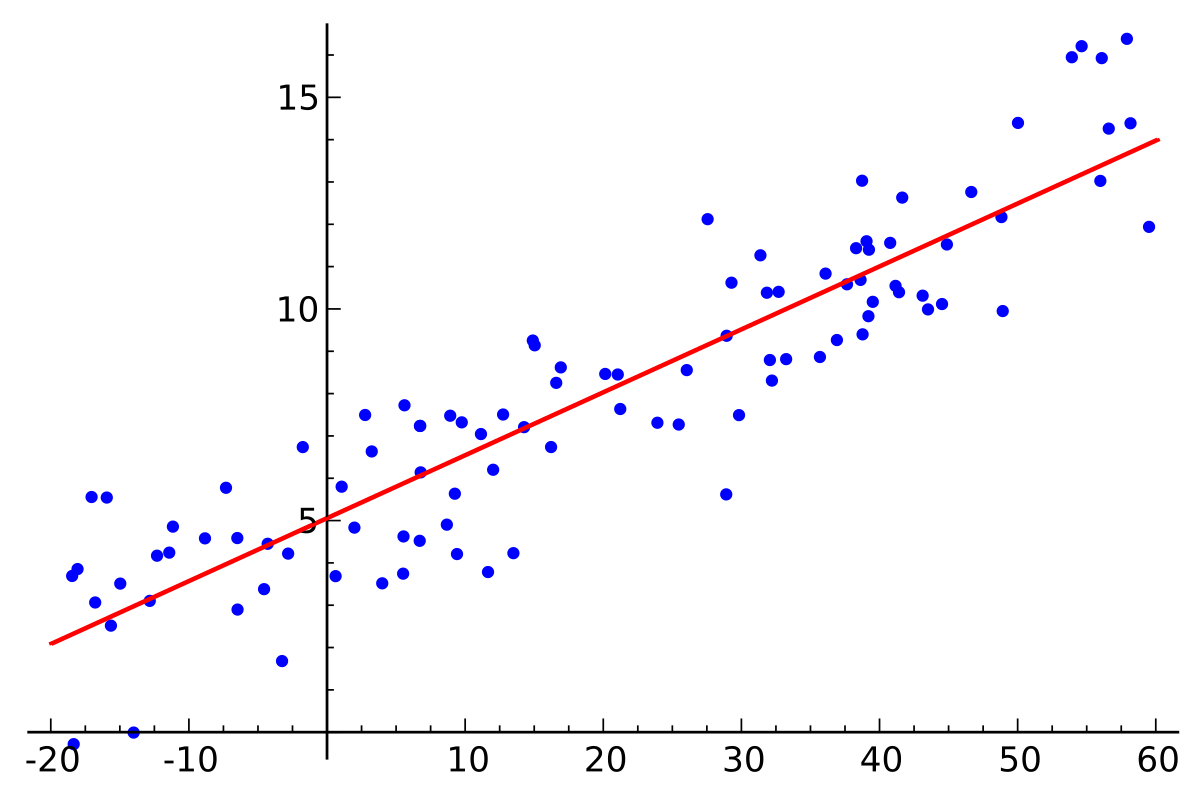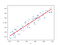# Linear RegressionLinear Regression

Linear Regression is one of the most fundamental, yet powerful algorithms used in Supervised Machine Learning. It is simple, easily understandable, and used for solving regression types of problems. In Linear Regression, we try to find the relationships between the independent variables (X) and dependent variable (y), and in the future, for unseen (X) it predicts (y). If only a single (X) is available, then it is called a simple linear regression, if more number of (X) variables are present, it is called a multiple linear regression.

The relation between X and y is nothing but a mathematical equation that tries to hold the relationship between X and y. Let us consider an example, where we have values of weights of a group of population corresponding to their heights. Say for heights 5.3 ft, 5.8 ft, 6.1 ft, 5.4 ft, 5.6 ft …. their respective weights are 62 kg, 65 kg, 68 kg, 66 kg, 67 kg … Here if there is a person with height of 6.2 ft, could we able to give his weight?? The human mind could guess some approx. numbers.. but how does a machine able to learn relationships from given data and predict it ??

By seeing the dataset, we could understand someway or other, weights are directly or inversely proportional to heights i.e, weights could be expressed in terms of height.

Weight (W) = 10.53(m)* Height (H) (+/-) error (C)

We could see above equation is similar to, equation of line y = mx + c. Let us plot the variables X and y in graphs for better visualization. Python gives us beautiful packages for visualization of datasets such as seaborn and matplotlib. Using scatterplots, we could see that the dataset follows a linear pattern, hence a machine can find the best fit line to establish mathematical relationships between the variables X and y.The best fit line passing through the dataset

Simple Linear regression is given by equation y = mx + c, where

y = dependent variable

x = independent variable

m = co-efficient of x/slope (unit change in y for a unit change in x)

c = error term / intercept

m’ and ‘c’, determine the best relation between the variables, hence it is a significant term for the linear model. m and c are estimated using least square criteria, i.e the best fit line which has a minimum sum of squared errors/residuals.

Assumptions of Linear Model:

• The dataset follows a linear pattern, so the model could be built with coefficients of x and intercept.
• Our aim is to find the relationship between the dependent and independent variables. Hence there should be no multicollinearity, i.e no correlation between the independent variables.
• The mean of residuals is zero.
• Homosceadasicity. Error terms have constant variance.
• Error terms are normally distributed.
• Error terms are not correlated among them, as well as not correlated with the independent variables.

Best fit line:

The best fit line is found by minimizing the residual, where residual is the distance between the output (y) and predicted (y^). A gradient-based approach could be followed for reducing loss/minimizing the residual to find the best fit line. Loss needs to be decreased by optimizing coefficients of the model i.e., m and c values.

m (new) = m(old) — n(learning rate) * (de/dm) differentiate error(e) w.r.t m

c (new) = c(old) — n(learning rate) * (de/dc)differentiate error(e) w.r.t c

Accuracy of the Model:

1. R-square statistics:

This measures the fitness of the line or accuracy of the regression model. It could be defined as one minus the ratio between Residual Sum of Square (RSS) and Total Sum of Square (TSS).

R-square = 1 - (RSS/TSS)

Where RSS = summation of y-(y^)

TSS = summation of y-(y_bar)

The value of R-square lies between 0 and 1. If the value is closer the 1, the better the model accuracy is. Say if the R-square value is 0.80, the model could explain 80% of the relation between the variables.

2. Adjusted R-square statistics:

As the number of independent variables (X) increases, the value of R-square also increases, which does not mean there is any correlation between new X and y. In other words, by the addition of new independent variables, the model accuracy doesn’t increase with an increase in R-square value. To rectify this, an Adjusted R-square is used, which penalizes the effect of additional variables X which has no relation with y.

Adjusted R-square = 1 — [(1-Rsquare)*(N-1)/(N-p-1)]

N = Total sample size

p = Number of independent variables